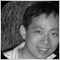# Need Help--whats wrong with my code?45

double a[];
for (int i=0; i<Bars; i++)
{
a[i] = (3*Close[i]+Open[i]+High[i]+Low[i])/3;
}
.........

what`s wrong with the code above?the a[i] seems get nothing?when I used Print() to check the a[i], I found a[i] is zero,why? can somebody help me ? Thanks!490

shawnyau:
double a[];
for (int i=0; i<Bars; i++)
{
a[i] = (3*Close[i]+Open[i]+High[i]+Low[i])/3;
}
.........

what`s wrong with the code above?the a[i] seems get nothing?when I used Print() to check the a[i], I found a[i] is zero,why? can somebody help me ? Thanks!
The size of a[] has to be defined, either a or whatever number, or use ArrayResize().2646

In this case:

double a[];

ArrayResize(a, Bars);

... because he is going to fill it with that many values. The array must be sized to hold the number of elements you plan to store.45

thank you!